# Circle section

Equilateral triangle with side 33 is inscribed circle section whose center is in one of the vertices of the triangle and the arc touches the opposite side.

Calculate:

a) the length of the arc
b) the ratio betewwn the circumference to the circle sector and the perimeter of the triangle

Correct result:

x =  29.9277 cm
y =  0.3023

#### Solution: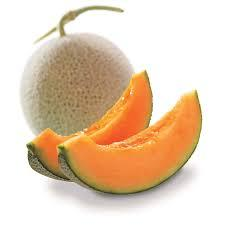We would be pleased if you find an error in the word problem, spelling mistakes, or inaccuracies and send it to us. Thank you!Tips to related online calculators
Pythagorean theorem is the base for the right triangle calculator.

#### You need to know the following knowledge to solve this word math problem:

We encourage you to watch this tutorial video on this math problem:

## Next similar math problems:

• Center traverseIt is true that the middle traverse bisects the triangle?
• Reference angleFind the reference angle of each angle:The double ladder shoulders should be 3 meters long. What height will the upper top of the ladder reach if the lower ends are 1.8 meters apart?The double ladder is 8.5m long. It is built so that its lower ends are 3.5 meters apart. How high does the upper end of the ladder reach?
• Horizon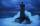The top of a lighthouse is 19 m above the sea. How far away is an object which is just “on the horizon”? [Assume the earth is a sphere of radius 6378.1 km.]
• ThalesCalculate the length of the Thales' circle described to right triangle with hypotenuse 44.2 cm.
• Circle inscribed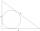Calculate the perimeter and area of a circle inscribed in a triangle measuring 3 , 4 and 5 cm.
• Right angleIf a, b and c are two sides of a triangle ABC, a right angle in A, find the value on each missing side. If b=10, c=6
• Circles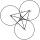Three circles of radius 95 cm 78 cm and 64 cm is mutually tangent. What is the perimeter of the triangle whose vertices are centers of the circles?
• Circumscribing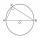Determine the radius of the circumscribed circle to the right triangle with legs 9 cm and 6 cm.
• Equilateral triangleHow long should be the minimum radius of the circular plate to be cut equilateral triangle with side 19 cm from it?
• Perimeter of circleCalculate the circumference of described circle to the triangle with sides 9,12,15 cm.
• MedianIn triangle ABC is given side a=10 cm and median ta= 13 cm and angle gamma 90°. Calculate length of the median tb.
• RT - inscribed circleIn a rectangular triangle has sides lengths> a = 30cm, b = 12.5cm. The right angle is at the vertex C. Calculate the radius of the inscribed circle.
• Pendulum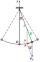Calculate the length of the pendulum that is 2 cm lower in the lowest position than in the highest position. The length of the circular arc to be described when moving is 20cm.
• Field with vegetables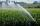Field planted with vegetables has shape of a rectangular isosceles triangle with leg length of 24 m. At the vertices of the triangle are positioned rotating sprinklers with a range of 12 m. How much of the field sprinkler doesn't irrigated?
• Perimeter of triangleIn triangle ABC angle A is 60° angle B is 90° side size c is 15 cm. Calculate the triangle circumference.